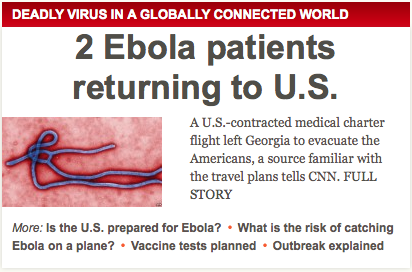## Thursday, July 31, 2014

### 33 44 | The Ebola Scare Is Coming to GeorgiaEbola = 5+2+6+3+1 = 17; think MH-17
The Ebola scare is coming to the United States of America, and it is set to take place in Georgia.  Let us recall that the 'Ebola Scare Story' began with a 33-year old patient zero, much like the 33-year old doctor who discovered Michael, Patient Zero for AIDS.
• 33-year old sick with Ebola
• 33-year old doctor discovered Patient Zero for AIDS
• AIDS = 1+9+4+19 = 33
• AIDS patient is named 'Michael' and is 31-years old
• Michael = 4+9+3+8+1+5+3 = 33
• 31-33 are the ranging Degrees of Freemasonry
• Variola is what wiped out the 'Indian'
• Variola = 4+1+9+9+6+3+1 = 33
• Indian = 9+5+4+9+1+5 = 33
In the early days of AIDS awareness, it was associated with being 'Gay' or 'Homosexual'.  As we decode those words, think about their associations with "queer and happy".
• Gay = 7+1+25 = 33
• Homosexual = 8+15+13+15+19+5+24+21+1+12 = 133
• Queer = 17+21+5+5+18 = 66
• Happy = 8+1+16+16+25 = 66
• Freedom = 6+18+5+5+4+15+13 = 66
• Thirty-Three = 2+8+9+9+2+7+2+8+9+5+5 = 66
On the subject of numerology and sickness, let us also acknowledge the Gematria of the words 'sick' and 'ill'.
• Sick = 19+9+3+11 = 42
• Ill = 9+12+12 = 33
• These numbers are often paired together, in names from Patrick to Kennedy to Knight
Anyhow, if they ever decide to wipe out the population of the United States, I believe it will begin in the Georgie region, where the Georgia Guidestones are located, which warn of drastically decreasing the earth's population to 500-million.  Further, Georgia is the 4th State with a Gematria of "44", which is associated with death, destruction and assassination.
• Georgia = 7+5+6+9+7+9+1 = 44
• Georgia is the 4th State
• Books and movies titled 444 deal with the end of the world
• There have been many assassination on 4/4; from MLK to John Henry Harrison.
• Currently, Barack Hussein Obama is the 44th President
What does it all mean?  In time, we shall see.  Truth be told, I honestly think we could use a good cleansing.  Most people think their worth is nill and the best they can do it go along with the system they hate.  You know it and I know it.  When you think about it, you see just how worthless of an existence that is.  So why not cleanse it?  What are these people of this earth if nothing more than the most destructive force against all other things living?  The truth hurts, but that is what this blog is about.

Let us close by looking at where the plane picking up the Ebola victims left from, Cartersville, Georgia.
• Cartersville = 3+1+9+2+5+9+1+22+9+3+3+5 = 72
• Georgia = 7+5+6+9+7+9+1 = 44
• Cartersville, Georgia = 72+44 = 116
Notice that the number 116 is the number 911 upside down.  The following names and phrases all have Gematria of 116
• Meaning of Life
• Eternity
• Tony Blair
• Goldman Sachs
• Illuminate
• Nuclear War
• and many, many more...
• ...it isn't just words into names either...
• \$116-million was at the center of the BitCoin scandal
• A bomb went off at 116th Street in New York City earlier this year
With regards to 911, the date September 11 is New Year's Day on the Coptic Calendar, which is the old world calendar the Egyptians went by.  Who knew?
• Coptic = 3+15+16+9+20+9+3 = 66
• Thirty-Three = 2+8+9+9+2+7+2+8+9+5+5 = 66
• The sum of the first 13-Colonies is "66"
• The first highway across the nation was Route 66
• In 66 AD, the Jewish Revolt against Rome occurred
One last important point, the outbreak for Ebola is said to be in 'Conakry' in Guinea.  Conakry has "33" gematria.  This is the same place the "33-year old" doctor became infected.

• Conakry = 3+6+5+1+2+9+7 = 33

1.Very interesting...many people disregard numerology, but there's some truth to it.

2.Hi Lady Danger, you should investigate Gematria and Pythagoras. You'll come to understand that the English alphabet being of "26" letters is no accident. In Gematria "26" is the "God Number"
God = 7+15+4 = 26.
You can also read this post of mine on the origins of the English language. http://freetofindtruth.blogspot.com/2014/07/33-74-robert-wylkynson-and-english.html

3.This comment has been removed by a blog administrator.